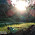# GATE Questions-Data Structures-Hashing

Previous GATE questions with solutions on Data Structures (Hashing) - CS/IT

GATE-2010
1. A hash table of length 10 uses open addressing with hash function h(k)=k mod 10, and linear probing. After inserting 6 values into an empty hash table, the table is as shown below.
 0 1 2 42 3 23 4 34 5 52 6 46 7 33 8 9 10

Which one of the following choices gives a possible order in which the key values could have been inserted in the table?
(a) 46, 42, 34, 52, 23, 33
(b) 34, 42, 23, 52, 33, 46
(c) 46, 34, 42, 23, 52, 33
(d) 42, 46, 33, 23, 34, 52

Ans: option (c)
Explanation:
Linear Probing - The location where the key value should be stored in the table is determined by the hash function. Here hash function is k mod 10. For e.g., if we need to store 42 then find 42 mod 10, then we get the value 2, that means the key value 42 should be stored in the table index 2. When there is a collision, linearly come down to locate next empty location and store the value in that location.
For the above question, only option (d) will give you the above hash table. Hash table for all options are given below:
 A B C D 0 1 2 42 42 42 42 3 52 23 23 33 4 34 34 34 23 5 23 52 52 34 6 46 33 46 46 7 33 46 33 52 8 9 10

GATE-2010
2. How many different insertion sequences of the key values using the same hash function and linear probing will result in the hash table shown above?
(a) 10    (b) 20    (c) 30    (d) 40

Ans: option (c)
Explanation:
For the above hash table to occur, collision occurs for 52 and 33. That means, 42 and 23 must always appear before 52 and 33. Also note that 34 should always come before 52 and 33, else 52 and 33 may occupy 34's place. Therefore 42,23, and 34 should appear before 52 and 33. Hence 42, 23 and 34 can occur in 3! ways.
Now notice that 52 should come before, and finally 46 can appear at 5 different places.

Therefore, total number of different sequences = 3! x 5 = 30

GATE-2004
3. Given the following input (4322, 1334, 1471, 9679, 1989, 6171, 6173, 4199) and the hash function x mod 10, which of the following statements are true?
i) 9679, 1989, 4199 hash to the same value
ii) 1471, 6171 has to the same value
iii) All elements hash to the same value
iv) Each element hashes to a different value
(a) i only
(b) ii only
(c) i and ii only
(d) iii or iv

Ans: option (c)
Explanation:
Refer question number 1.

GATE-2007
4. Consider a hash table of size seven, with starting index zero, and a hash function (3x + 4) mod 7. Assuming the hash table is initially empty, which of the following is the contents of the table when the sequence 1, 3, 8, 10 is inserted into the table using closed hashing? Note that ‘_’ denotes an empty location in the table.
(a) 8, _, _, _, _, _, 10
(b) 1, 8, 10, _, _, _, 3
(c) 1, _, _, _, _, _,3
(d) 1, 10, 8, _, _, _, 3

Ans: option (b)
Explanation:
Refer question number 1.
h(x) = (3x + 4) mod 7
h(1) = (3 + 4) mod 7 = 0
h(3) = (9 + 4) mod 7 = 6
h(8) = (24 + 4) mod 7 = 0
h(10) = (3 + 4) mod 7 = 6

 0 1 1 8 2 10 3 4 5 6 3

#### 1 comment:

1.Explanation seems not correct, the correct answer will (c) as closed hashing has been used here. In case of open hashing (b) is correct.